January 2016How can you use control charts with a process where you expect the average response to trend up or trend down over time?  Last month we took a look at global warming through the eyes of a control chart.

The data indicated that there were periods of stability – where the temperature neither increased or decreased – until around 1997.  Since then, there appears to have been a steady upward trend in the global temperature on almost a yearly basis.

Does this mean that we can’t use control charts anymore?  No, not at all.  In fact, we used a trend control chart last month to monitor the data from 1997 on.  In this month’s publication, we will examine how you construct and interpret a trend control chart using a slightly different set of global temperature data.

In this issue:

### Classical Shewhart Control ChartsThe classical Shewhart control chart consists of two control charts.  Examples of these classical control charts include the X-R and individuals (X-mR) control charts.  With the individuals control chart, one chart (X Chart) monitors the variation “between” individual results.  The second chart monitors the variation between consecutive results (mR chart).  This is the “within” variation.  One objective of this control chart is to determine if the process is in statistical control.

If it is in statistical control, we typically think that the process has one average and one measure of variation.  The “one” average comes from the center line on the X chart.  The measure of variation (the estimated standard deviation) comes from the average range on the mR chart.   Of course, no process is unwavering, but it is helpful to think about statistical control, in the classical sense, as having one average and one measure of variation.  This is shown in Figure 1.  But what about processes where you don’t expect there to be just “one” average?  That is where the trend control chart comes in.

Figure 1: In Control Process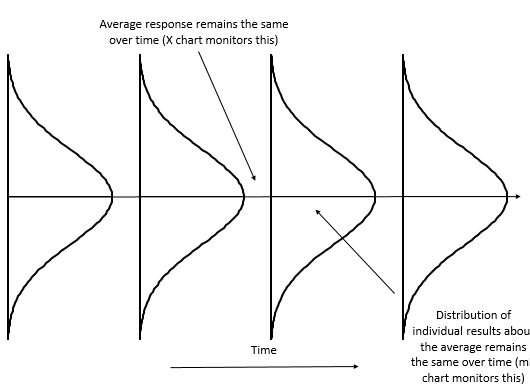### Trend Control Charts

There are processes where you don’t expect the average to stay the same over time.  You expect the average to move up or down.  Examples include catalyst activity, financial items affected by inflation, and tool wear.  If these parameters are plotted using the classical Shewhart control charts, the process will appear out of control because the average is not staying the same.  But the process is not out of control because the average naturally moves up or down over time.  This is shown in Figure 2.

Figure 2: Variation in a Process with a Trend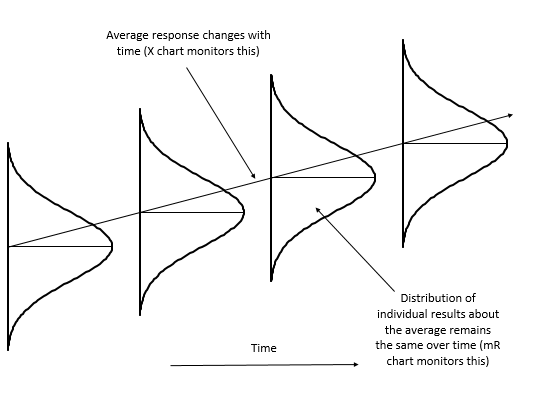Trend control charts provide a method of handling this type of situation.  A trend control chart still consists of two charts.  One is the moving range chart.  This is identical to the moving range used in the classical Shewhart control charts.  The other is the X chart, but in this X chart, the average is not horizontal but moves up or down.  The average represents the best-fit line for the data.  The process for creating a trend control chart is shown below using the global temperature data.

### Global Temperature DataThe data used in this analysis was downloaded from the Goddard Institute for Space Studies (GISS) website at this link.  GISS is part of the National Aeronautics and Space Administration (NASA).  Last month, we looked at using a control chart to see periods of stability and periods when the global temperature was increasing.  The data covered the years from 1880 – 2015.   The annual data was used in last month’s publication.  The data were based on the meteorological station data, which is called the dTs data.

There is also the LOTI (land-ocean temperature index).  According to the website, LOTI "provides a more realistic representation of the global mean trends than dTs; it slightly underestimates warming or cooling trends, since the much larger heat capacity of water compared to air causes a slower and diminished reaction to changes; dTs on the other hand overestimates trends, since it disregards most of the dampening effects of the oceans that cover about two thirds of the earth's surface."

This month we will use the LOTI data.  It, like the dTs data, is reported as anomalies (deviations), not an absolute temperature.  The website has this statement as well: “Temperature anomalies are computed relative to the base period 1951-1980. The reason to work with anomalies, rather than absolute temperature, is that absolute temperature varies markedly in short distances, while monthly or annual temperature anomalies are representative of a much larger region.”  In last month’s publication, we converted these anomalies from the baseline period to an absolute temperature by using 14 °C as the baseline temperature.  It is sometimes easier to understand an absolute temperature than the anomalies.  But 14 is not absolute.  Like everything, there is variation.

This time, however, we will use the anomalies as given on the website.  But, we will refer them as deviations from here on.    For example, for 2015, the deviation in temperature from the baseline period was reported as 87.  You divide this by 100 to get the deviations in degrees Celsius.  So, the deviation in 2015 from the baseline period was 87/100 or 0.87 °C.   According to the press release on 1/20/2016, this is the largest increase in one year ever.

Our analysis last month showed that there was a long period of stability from 1936 to 1976.  The temperature then began to increase in 1977.  We will use the data from 1977 to 2015 in our trend control chart discussion.  These data are shown in Table 1.

Table 1: Temperature Deviations Based on Baseline Period of 1951 to 1980

 Year Deviation Year Deviation 1977 18 1997 47 1978 7 1998 63 1979 17 1999 42 1980 28 2000 42 1981 33 2001 54 1982 13 2002 63 1983 31 2003 62 1984 16 2004 54 1985 12 2005 69 1986 19 2006 63 1987 34 2007 66 1988 40 2008 53 1989 29 2009 64 1990 44 2010 72 1991 42 2011 60 1992 23 2012 63 1993 24 2013 65 1994 32 2014 74 1995 46 2015 87 1996 34

### Classical Control Chart Approach

The data in Table 1 were used to construct an individuals control chart.   The X control chart for the data is shown in Figure 3.  You can easily see that the global temperature deviation is not consistent over time.  It appears to be steadily increasing.  There are runs above and below the average as well as points that are beyond the control limits.  This X control chart is not very useful for predicting what will occur in the future.

Figure 3: X Chart for Global Temperature Deviations by Year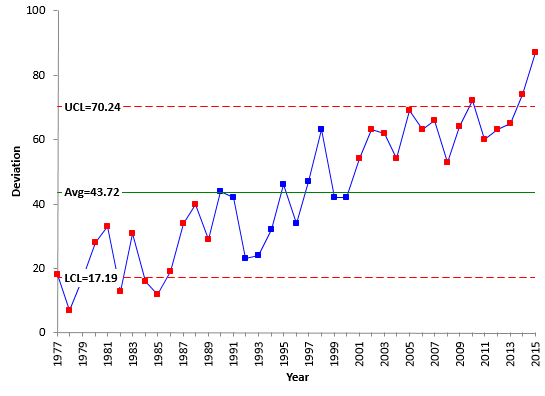Figure 4 shows the moving range control chart.  The moving range is in statistical control.  It is consistent and predictable.  This means, that even though the average is changing over time, the variation about that average (the width of the histogram) is staying the same.  As long as this is true, we can use the trend control chart approach because the within variation (the moving range) is consistent over time.

Figure 4: mR Chart of Global Temperature Deviation by Year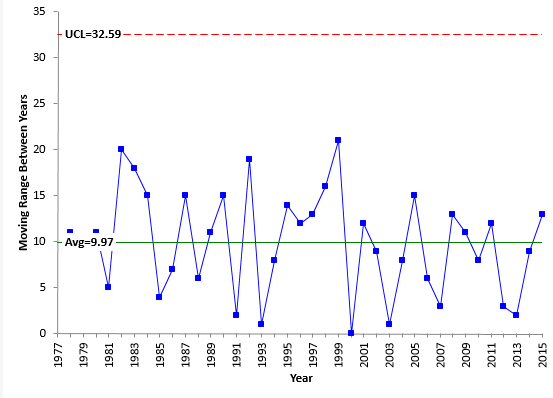The problem is the X control chart.   The control limits for the X control chart are given below.

UCLx = X + 2.66R

LCLx = X - 2.66R

Note that the control limits are based on the overall average (X) and the average range (R).  The problem is the average in the global temperature deviations. It looks to be increasing in a predictable pattern.  Let’s check this with the trend control chart.

### Trend Control Chart ApproachThe trend control chart will help handle situations where the data are increasing or decreasing in a predictable fashion.  The first step is to determine the best-fit line of the data.  This equation has the following form:

Xt = mt + b

where (for our example) Xt is yearly temperature deviation, t is the year, m is the slope of the line and b is the y-intercept.  You then substitute this equation for the average in the control limit equations.  So, the control limits for the trend control chart are given by:

UCLx = mt + b + 2.66R

LCLx = mt + b - 2.66R

There are a number of ways to find the best-fit equation.  The results below are from the SPC for Excel program.  You can also use Excel’s built-in regression function.  The best-fit equation is:

Global Temperature Deviation = 1.631(Year) - 3211.3

The slope represents the rate of change in the global temperature deviation per year for this time period.   That rate of change is given by 1.631.  Remember that to convert this to degrees Celsius, you need to divide by 100.  So the rate of change is 0.01631 °C per year.

You must ask a question at this point about this best-fit equation.  “Is it statistically significant?"  You judge this by the p-value from the regression.  If the p-value is less than 0.05, you conclude it is statistically significant.  The p-value for this regression is much lower than 0.05, so it is statistically significant.  For more information on regression, please see our publication at this link.

One more questions to ask.  “Is the statistically significant correlation between the global temperature deviation and the year “worth” anything in real life?”  You get this from the R-squared value.  R-squared for this regression is 82.37%, which means that 82.37% of the variation in global temperature deviation can be explained by the increasing year number.  That is a pretty good percentage.  So, this best-fit equation can be used to help analyze the increase in global temperature deviation from year to year.

The control limits become:

UCLx = b + mt + 2.66R = -3211.3 + 1.631(Year) + 2.66(10)

LCLx = b + mt - 2.66R = -3211.3 + 1.631(Year) – 2.66(10)

Note that the control limits will trend with the average and the distance between the average and the control limits will be 2.66 times R.  Figure 5 shows the trend control chart for the global temperature deviation.

Figure 5: Trend Control Chart for Global Temperature Deviation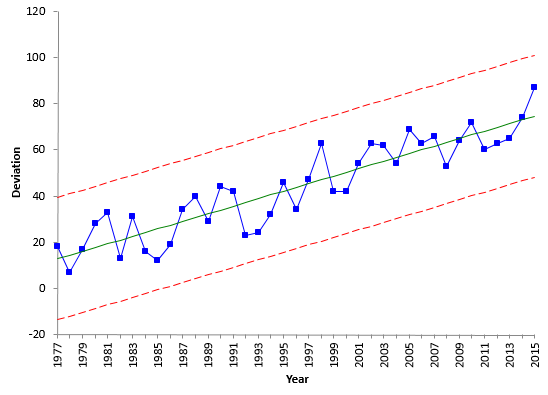You can use the best-fit equation to determine the values for the average, UCL and LCL in 2015.

UCL = 101.8

Average = 75.2

LCL = 48.6

When you look at the trend control chart, it appears to be in statistical control.  The usual out of control tests apply to the trend control chart – points beyond the limits, zones tests, etc.

You can now use the trend control chart to predict, within a range, what the deviation in the global temperature will be in 2016.

UCL = 103.4

2016 Prediction = 76.8

LCL = 50.2This “model” predicts that the 2016 results will be 76.8 and in between 50.2 and 103.4.  Remember that the control limits are set at +/- three standard deviations, so there is a “wide”” range of possible results.  Most the time, the results will be within +/1 one standard deviation which would place the 2016 result from about 70 to 86.

The trend control chart performs just like a classical control chart.  It monitors the process for any special causes of variation and, if it is in statistical control, it can be used to predict future values- at least, within a range.

### Summary

This month we took a look at how to handle data that is increasing or decreasing in a predictable fashion using a trend control chart.  The calculations involve determining the best-fit line for the data and using that as the center line on the X chart.  The center line and the control limits trend either up or down on a trend control chart.  The global temperature deviation per year was used as an example.

SPC for Excel Software

SPC Training

SPC Consulting

Ordering Information

Thanks so much for reading our publication. We hope you find it informative and useful. Happy charting and may the data always support your position.

Sincerely,

Dr. Bill McNeese
BPI Consulting, LLC#### Connect with Us

•You deserve my Congratulations for your (pedagogical) work.I suggest you illustrate this and other examples with your software. You may also provide a trial/student version. If so, I can use it, and promote your software, in my classes.Best regards

Jan 31, 2016
•Thanks for your comment.   All the charts in our publications are made using the SPC for Excel software.  And we do have free 20-day demo of the software here:https://www.spcforexcel.com/spc-software/demo

We do a studenet version that can be rented for 6 monhts.  Please contact me if you interested.

Bill

Jan 31, 2016
•This sentence, "R-squared for this regression is 82.37%, which means that 82.37% of the variation in global temperature deviation can be explained by the increasing year number", I suggest is better stated as," ...82.37% of the variation in gobal temperature deviation is accounted for by the increasing year number."Isn't R-squared an accounting? Or are accounting and explaining synonyms?

Jul 18, 2017
•I think, in this case, they are synonyms.  But I like your sentence better.

Jul 18, 2017
•Hello,i am trying to create a Trend Control chart and was using your above example to replicate the process. I am new to control chart and was hoping if someone could explain how the Upper and lower limit was calculated for the above trend control chart.As stated above, the UCL is calculated using UCLx = b + mt + 2.66 = -3211.3 + 1.631(Year) + 2.66(10) (here,y-intercept has a value of -3211.3, given the y-intercept has such a low value does that not make the UCLx =3301.509 for 2015??  if the t = 39 (3211.3+1.631*(39)+2.66*(10)) Could you also explain how the R for the above example is 10.your response will be much appreicated.

May 25, 2019
•The SPC for Excel software, when calculating a trend, assumes the x values are 1, 2, 3, 4, etc.  Not the actual values used (in this exmaple the years).  So the equation will be different since I used excel's regression to use the acutal  year number and not 1, 2, 3, etc.).  Probably need to change that.   Rbar is 10.  It is the average range for the moving ranges between consecutive points.

May 25, 2019

### Filtered HTML

• Allowed HTML tags: <a> <em> <strong> <cite> <code> <ul> <ol> <li> <dl> <dt> <dd> <h1> <h2> <h3> <h4> <h5> <h6> <img> <hr> <div> <span> <strike> <b> <i> <u> <table> <tbody> <tr> <td> <th>
• Lines and paragraphs break automatically.

### Plain text

• No HTML tags allowed.
• Lines and paragraphs break automatically.
This question is for testing whether you are a human visitor and to prevent automated spam submissions.

#### SPC Knowledge BaseSign up for our FREE monthly publication featuring SPC techniques and other statistical topics.